## Example Questions

1 2 9 10 11 12 13 14 15 17 Next →

### Example Question #161 : Percentage

In business, profit is defined as revenue minus expenses. A profit is often expressed as a percentage of total revenue.

A company posts a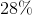profit for the first quarter of the new year, pleasing investors. If the total profits for the quarter were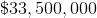what were the expenses for the company in that quarter? Round to the nearest dollar.

Possible Answers: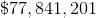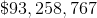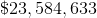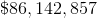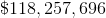Correct answer:Explanation:

If a profit ofrepresents, then we can calculate revenue as: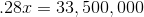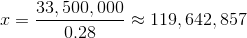Now, subtract the profit from the total revenue to calculate expenses: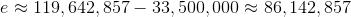Thus, the quarterly expenses were.

### Example Question #12 : How To Find Amount Of Profit

Manufacturing a particular product produces a profit of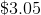for each unit produced. If the cost to produce a unit is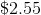, what is the profit percentage on each unit? Do not round until the final answer.

Possible Answers: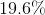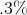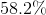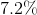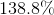Correct answer:Explanation:

Calculating a percentage profit is the same as calculating a percent change. To find percent change, subtract the "old" amount from the "new" amount, then divide the result by the "old" amount. The result is a percent increase if positive and a percent decrease if negative.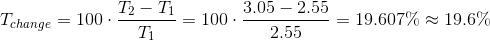### Example Question #13 : How To Find Amount Of Profit

A particular product is sold for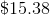per unit. If the cost to produce a unit is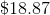, what is the profit percentage on each unit? Do not round until your the answer.

Possible Answers: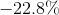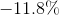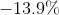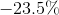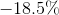Correct answer:Explanation:

Calculating a percentage profit is the same as calculating a percent change. To find percent change, subtract the "old" amount from the "new" amount, then divide the result by the "old" amount. The result is a percent increase if positive and a percent decrease if negative.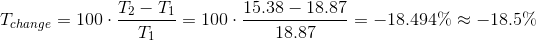### Example Question #251 : Arithmetic

A cassette tape sells for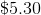, and the producer receives a cut ofof each sale. If the producer spends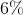of the sale price in manufacturing and producing expenses, how much money is made on the sale of each tape?

Possible Answers: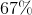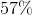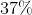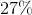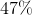Correct answer:Explanation:

Calculating a percentage profit is the same as calculating a percent change. To find percent change, subtract the "old" amount from the "new" amount, then divide the result by the "old" amount. The result is a percent increase if positive and a percent decrease if negative.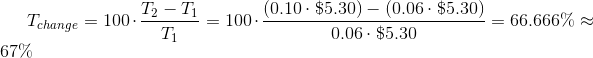### Example Question #11 : How To Find Amount Of Profit

A product sells for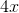dollars, and costs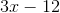dollars to produce. What is the profit on each sale, as a percentage?

Possible Answers: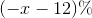There is not enough information to answer the question.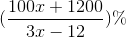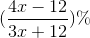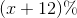Correct answer:Explanation:

Calculating a percentage profit is the same as calculating a percent change. To find percent change, subtract the "old" amount from the "new" amount, then divide the result by the "old" amount. The result is a percent increase if positive and a percent decrease if negative.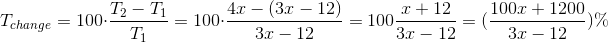1 2 9 10 11 12 13 14 15 17 Next →

### All ACT Math Resources